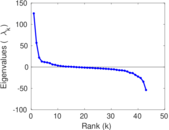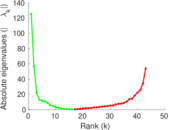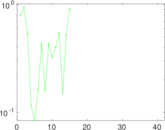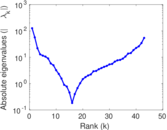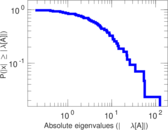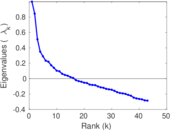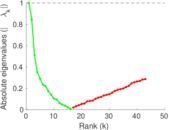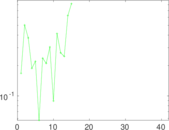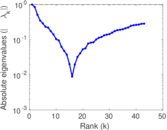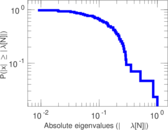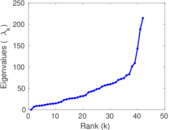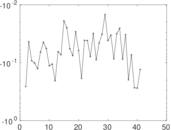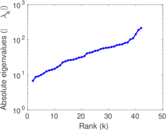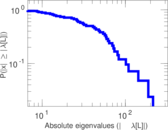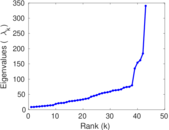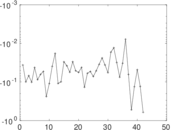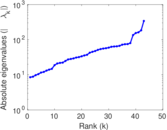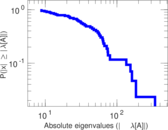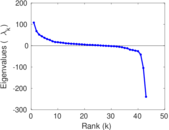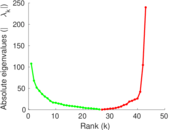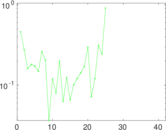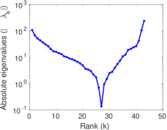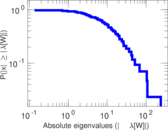# Windsurfers

This undirected network contains interpersonal contacts between windsurfers in southern California during the fall of 1986. A node represents a windsurfer and an edge between two windsurfers shows that there was a interpersonal contact.

 Code `MW` Internal name `moreno_beach` Name Windsurfers Data source http://moreno.ss.uci.edu/data.html#beach AvailabilityDataset is available for download Consistency checkDataset passed all tests Category Human contact network Node meaning Person Edge meaning Contact Network format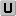Unipartite, undirected Edge type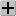Positive weights, no multiple edges LoopsDoes not contain loops

## Statistics

 Size n = 43 Volume m = 336 Loop count l = 0 Wedge count s = 5,831 Claw count z = 36,530 Cross count x = 180,422 Triangle count t = 1,096 Square count q = 12,034 4-Tour count T4 = 120,268 Maximum degree dmax = 31 Average degree d = 15.627 9 Fill p = 0.372 093 Size of LCC N = 43 Diameter δ = 3 50-Percentile effective diameter δ0.5 = 1.265 55 90-Percentile effective diameter δ0.9 = 1.926 56 Median distance δM = 2 Mean distance δm = 1.696 51 Gini coefficient G = 0.237 403 Balanced inequality ratio P = 0.409 226 Relative edge distribution entropy Her = 0.976 716 Power law exponent γ = 2.157 90 Tail power law exponent γt = 5.021 00 Tail power law exponent with p γ3 = 5.021 00 p-value p = 0.286 000 Degree assortativity ρ = −0.146 965 Degree assortativity p-value pρ = 0.000 131 592 Clustering coefficient c = 0.563 883 Spectral norm α = 125.530 Algebraic connectivity a = 6.697 93 Spectral separation |λ1[A] / λ2[A]| = 2.219 41 Non-bipartivity bA = 0.724 591 Normalized non-bipartivity bN = 0.678 918 Algebraic non-bipartivity χ = 5.586 32 Spectral bipartite frustration bK = 0.089 364 5 Controllability C = 0 Relative controllability Cr = 0.000 00

## Plots

### Fruchterman–Reingold graph drawing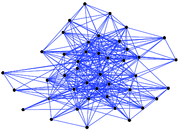### Degree distribution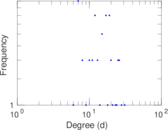### Cumulative degree distribution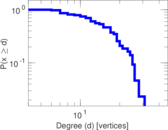### Lorenz curve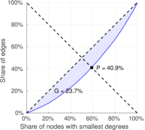### Spectral distribution of the adjacency matrix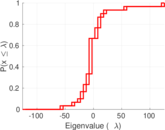### Spectral distribution of the normalized adjacency matrix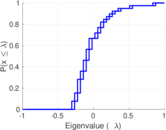### Spectral distribution of the Laplacian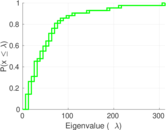### Spectral graph drawing based on the adjacency matrix### Spectral graph drawing based on the Laplacian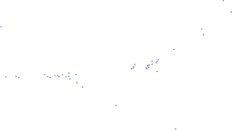### Spectral graph drawing based on the normalized adjacency matrix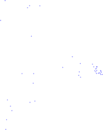### Degree assortativity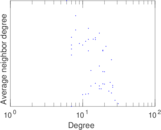### Zipf plot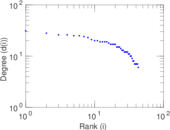### Hop distribution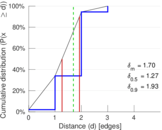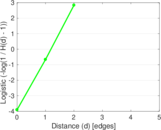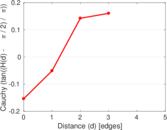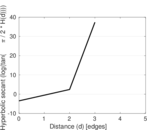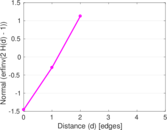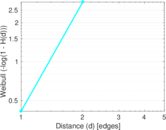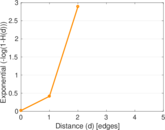### Double Laplacian graph drawing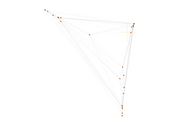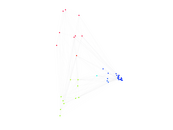### Delaunay graph drawing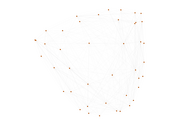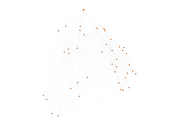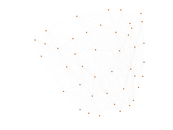### Edge weight/multiplicity distribution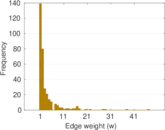### Clustering coefficient distribution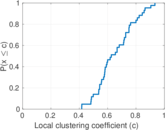### Average neighbor degree distribution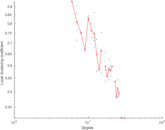### SynGraphy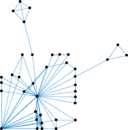### Matrix decompositions plots